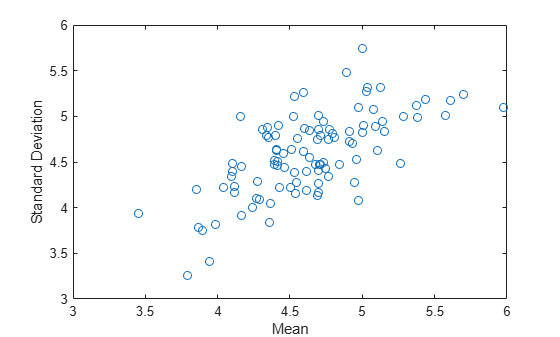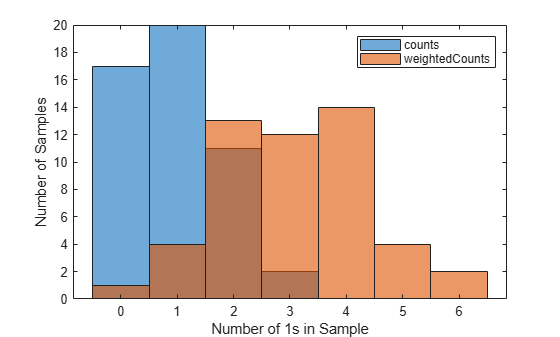bootstrp

Bootstrap sampling

Description

example

bootstat = bootstrp(nboot,bootfun,d) draws nboot bootstrap data samples from d, computes statistics on each sample using the function bootfun, and returns the results in bootstat. The bootstrp function creates each bootstrap sample by sampling with replacement from the rows of d. Each row of the output argument bootstat contains the results of applying bootfun to one bootstrap sample.

example

bootstat = bootstrp(nboot,bootfun,d1,...,dN) draws nboot bootstrap samples from the data in dl,...,dN. The nonscalar data arguments in dl,...,dN must have the same number of rows, n. The bootstrp function creates each bootstrap sample by sampling with replacement from the indices 1:n and selecting the corresponding rows of the nonscalar dl,...,dN. The function passes the sample of nonscalar data and the unchanged scalar data arguments in dl,...,dN to bootfun.

example

bootstat = bootstrp(___,Name,Value) specifies options using one or more name-value pair arguments in addition to any of the input argument combinations in previous syntaxes. For example, you can add observation weights to your data or compute bootstrap iterations in parallel.

example

[bootstat,bootsam] = bootstrp(___) also returns bootsam, an n-by-nboot matrix of bootstrap sample indices, where n is the number of rows in the original, nonscalar data. Each column in bootsam corresponds to one bootstrap sample and contains the row indices of the values drawn from the nonscalar data to create that sample.

To get the bootstrap sample indices without applying a function to the samples, set bootfun to empty ([]).

Examples

collapse all

Estimate the kernel density of bootstrapped means.

Generate 100 random numbers from the exponential distribution with mean 5.

rng('default')  % For reproducibility
y = exprnd(5,100,1);

Compute a sample of 100 bootstrapped means of random samples taken from the vector y.

m = bootstrp(100,@mean,y);

Plot an estimate of the density of the bootstrapped means.

[fi,xi] = ksdensity(m);
plot(xi,fi)Compute and plot the means and standard deviations of 100 bootstrap samples.

Generate 100 random numbers from the exponential distribution with mean 5.

rng('default')  % For reproducibility
y = exprnd(5,100,1);

Compute a sample of 100 bootstrapped means and standard deviations of random samples taken from the vector y.

stats = bootstrp(100,@(x)[mean(x) std(x)],y);

Plot the bootstrap estimate pairs.

plot(stats(:,1),stats(:,2),'o')
xlabel('Mean')
ylabel('Standard Deviation')Take bootstrap samples of patient data, compute the mean measurements for each data sample, and visualize the results.

Load the patients data set. Create the matrix patientData containing age, weight, and height measurements. Each row of patientData corresponds to one patient.

patientData = [Age Weight Height];

Create 200 bootstrap data samples from the data in patientData. To create each sample, randomly select with replacement 100 rows (that is, size(patientData,1)) from the rows in patientData. For each sample, calculate the mean age, weight, and height measurements. Each row of bootstat contains the three mean measurements for one bootstrap sample.

rng('default') % For reproducibility
bootstat = bootstrp(200,@mean,patientData);

Visualize the mean measurements for all 200 bootstrap data samples. Note that bootstrap samples with greater mean weights tend to have greater mean heights.

scatter3(bootstat(:,1),bootstat(:,2),bootstat(:,3))
xlabel('Mean Age')
ylabel('Mean Weight')
zlabel('Mean Height')

view([-75 10])Compute a correlation coefficient standard error using bootstrap resampling of the sample data.

Load the lawdata data set, which contains the LSAT score and law school GPA for 15 students.

rng('default')  % For reproducibility
size(lsat)
ans = 1×2

15     1

size(gpa)
ans = 1×2

15     1

Create 1000 data samples by resampling the 15 data points, and compute the correlation between the two variables for each data sample.

[bootstat,bootsam] = bootstrp(1000,@corr,lsat,gpa);

Display the first 5 bootstrapped correlation coefficients.

bootstat(1:5,:)
ans = 5×1

0.9874
0.4918
0.5459
0.8458
0.8959

Display the indices of the data selected for the first 5 bootstrap samples.

bootsam(:,1:5)
ans = 15×5

13     3    11     8    12
14     7     1     7     4
2    14     5    10     8
14    12     1    11    11
10    15     2    12    14
2    10    13     5    15
5     1    11    11     9
9    13     5    10     3
15    15    15     3     3
15    11     1     2     4
⋮

Create a histogram that shows the variation of the correlation coefficient across all the bootstrap samples.

histogram(bootstat)The sample minimum is positive, indicating that the relationship between LSAT score and GPA is not accidental.

Finally, compute a bootstrap standard of error for the estimated correlation coefficient.

se = std(bootstat)
se = 0.1285

Compare bootstrap samples with different observation weights. Create a custom function that computes statistics for each sample.

Create 50 bootstrap samples from the numbers 1 through 6. To create each sample, bootstrp randomly chooses with replacement from the numbers 1 through 6, six times. This process is similar to rolling a die six times. For each sample, the custom function countfun (shown at the end of this example) counts the number of 1s in the sample.

rng('default') %For reproducibility
counts = bootstrp(50,@countfun,(1:6)');

Note: If you use the live script file for this example, the countfun function is already included at the end of the file. Otherwise, you need to create this function at the end of your .m file or add it as a file on the MATLAB® path.

Create 50 bootstrap samples from the numbers 1 through 6, but assign different weights to the numbers. Each time bootstrp randomly chooses from the numbers 1 through 6, the probability of choosing a 1 is 0.5, the probability of choosing a 2 is 0.1, and so on. Again, countfun counts the number of 1s in each sample.

weights = [0.5 0.1 0.1 0.1 0.1 0.1]';
weightedCounts = bootstrp(50,@countfun,(1:6)','Weights',weights);

Compare the two sets of bootstrap samples by using histograms.

histogram(counts)
hold on
histogram(weightedCounts)
legend
xlabel('Number of 1s in Sample')
ylabel('Number of Samples')
hold offThe two sets of bootstrap samples have different distributions; in particular, the samples in the second set tend to contain more 1s. For example, of the 50 samples in the first set, only two samples contain more than two 1s. By contrast, of the 50 samples in the second set (with observation weights), $12+14+4+2=32$ samples contain more than two 1s.

This code creates the function countfun.

function numberofones = countfun(sample)
numberofones = sum(sample == 1);
end

Estimate the standard errors for a coefficient vector in a linear regression by bootstrapping the residuals.

Note: This example uses regress, which is useful when you simply need the coefficient estimates or residuals of a regression model and you need to repeat fitting a model multiple times, as in the case of bootstrapping. If you need to investigate a fitted regression model further, create a linear regression model object by using fitlm.

Perform a linear regression, and compute the residuals.

x = [ones(size(heat)),ingredients];
y = heat;
b = regress(y,x);
yfit = x*b;
resid = y - yfit;

Estimate the standard errors by bootstrapping the residuals.

se = std(bootstrp(1000,@(bootr)regress(yfit+bootr,x),resid))
se = 1×5

56.1752    0.5940    0.5815    0.5989    0.5691

Input Arguments

collapse all

Number of bootstrap samples to draw, specified as a positive integer scalar. To create each bootstrap sample, bootstrp randomly selects with replacement n out of the n rows of (nonscalar) data in d or d1,...,dN.

Example: 100

Data Types: single | double

Function to apply to each sample, specified as a function handle. The function can be a custom or built-in function. You must specify bootfun with the @ symbol.

For an example that uses a custom function, see Compare Bootstrap Samples with Different Observation Weights.

Example: @mean

Data Types: function_handle

Data to sample from, specified as a column vector or matrix. The n rows of d correspond to observations. When you use multiple data input arguments d1,...,dN, you can specify some arguments as scalar values, but all nonscalar arguments must have the same number of rows.

If you use a single vector argument d, you can specify it as a row vector. bootstrp then samples from the elements of the vector.

Data Types: single | double | logical | char | string | cell | categorical

Name-Value Arguments

Specify optional comma-separated pairs of Name,Value arguments. Name is the argument name and Value is the corresponding value. Name must appear inside quotes. You can specify several name and value pair arguments in any order as Name1,Value1,...,NameN,ValueN.

Example: bootstrp(4,@mean,(1:2)','Weights',[0.4 0.6]') specifies to draw four bootstrap samples from the values 1 and 2 and take the mean of each sample. For each draw, the probability of getting a 1 is 0.4, and the probability of getting a 2 is 0.6.

Observation weights, specified as the comma-separated pair consisting of 'Weights' and a nonnegative vector with at least one positive element. The number of elements in Weights must be equal to the number of rows n in the data d or d1,...,dN. To obtain one bootstrap sample, bootstrp randomly selects with replacement n out of n rows of data using the weights as multinomial sampling probabilities.

Data Types: single | double

Options for computing bootstrap iterations in parallel and setting random numbers during the bootstrap sampling, specified as the comma-separated pair consisting of 'Options' and a structure. Create the Options structure with statset. This table lists the option fields and their values.

Field NameValueDefault
UseParallelSet this value to true to compute bootstrap iterations in parallel.false
UseSubstreams

Set this value to true to run computations in parallel in a reproducible manner.

To compute reproducibly, set Streams to a type that allows substreams: 'mlfg6331_64' or 'mrg32k3a'.

false
StreamsSpecify this value as a RandStream object or cell array of such objects. Use a single object except when the UseParallel value is true and the UseSubstreams value is false. In that case, use a cell array that has the same size as the parallel pool.If you do not specify Streams, then bootstrp uses the default stream or streams.

Note

You need Parallel Computing Toolbox™ to run computations in parallel.

Example: 'Options',statset('UseParallel',true)

Data Types: struct

Output Arguments

collapse all

Bootstrap sample statistics, returned as a column vector or matrix with nboot rows. The ith row of bootstat corresponds to the results of applying bootfun to the ith bootstrap sample. If bootfun returns a matrix or array, then the bootstrp function first converts this output to a row vector before storing it in bootstat.

Bootstrap sample indices, returned as an n-by-nboot numeric matrix, where n is the number of rows in the original, nonscalar data. Each column in bootsam corresponds to one bootstrap sample and contains the row indices of the values drawn from the nonscalar data to create that sample.

For example, if each data input argument in d1,...,dN contains 16 values, and nboot = 4, then bootsam is a 16-by-4 matrix. The first column contains the indices of the 16 values drawn from d1,...,dN for the first bootstrap sample, the second column contains the indices for the second bootstrap sample, and so on. The bootstrap indices are the same for all input data sets d1,...,dN.

Tips

• To get the bootstrap sample indices bootsam without applying a function to the samples, set bootfun to empty ([]).